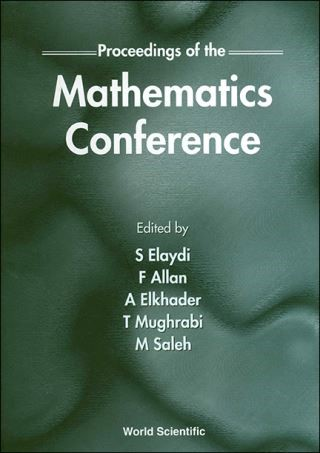## Proceedings Of The Mathematics Conference• Description
• Author
• Info
• Reviews

### Description

This volume covers topics ranging from pure and applied mathematics to pedagogical issues in mathematics. There are papers in mathematical biology, differential equations, difference equations, dynamical systems, orthogonal polynomials, topology, calculus reform, algebra, and numerical analysis. Most of the papers include new, interesting results that are at the cutting edge of the respective subjects. However, there are some papers of an expository nature.